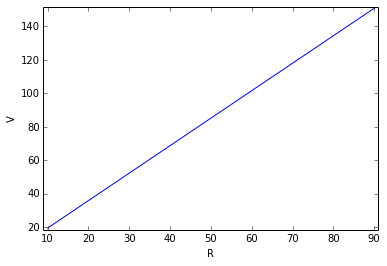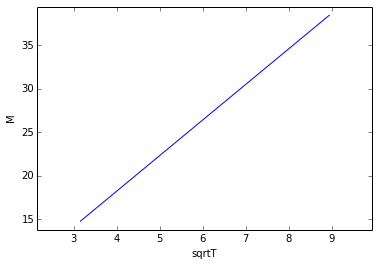# Chapter 2: Introduction to Engineering Calculations¶

## Example 2.2-1, page no. 10¶

In :
#Initialization of variables
import math
import numpy
from numpy import linalg

AcclInitial=1.0           #cm/s^2

#Calculations and printing :
print(" All the values in the textbook are Approximated hence the values in this code differ from those of Textbook")
AcclFinal=AcclInitial*math.pow(3600*24*365,2)/math.pow(10,5)
#the calculations involved are the conversion factors
print '%s %.3E' %(" \n final acceleration (Km/Yr^2) = ",AcclFinal)
raw_input('press enter key to exit')

 All the values in the textbook are Approximated hence the values in this code differ from those of Textbook

final acceleration (Km/Yr^2) =  9.945E+09
press enter key to exit

Out:
''

## Example 2.3-1, page no. 11¶

In :
#Initialization of variables
import math
import numpy
from numpy import linalg

Initial=23.0  #lb.ft/min^2

#Calculations and printing :

Final=Initial*0.453593*100/(3.281*60*60)
#the calculations involved are conversion factors
print '%s %.4f' %("final (kg.cm/s^2) = ",Final)
raw_input('press enter key to exit')

final (kg.cm/s^2) =  0.0883
press enter key to exit

Out:
''

## Example 2.4-1, page no. 13¶

In :
#Initialization of variables
import math
import numpy
from numpy import linalg

density=62.4            #lbm/ft^3
volume=2.0              #ft^3

#Calculations and printing :
mass=volume*density
print '%s %.3f' %("mass of the water = volume x density(lbm) = ",mass)
print(" \n At sealevel, g=32.174 ft/s^2")
g=32.174
weight=mass*g/32.174
print '%s %.3f' %(" \n weight at sealevel (lbf) = ",weight)
print(" \n At denver, g=32.139 ft/s^2")
g=32.139
weight=mass*g/32.174
print '%s %.3f' %("\n weight at denver (lbf) = ",weight)
#the division with 32.174 is to convert lbm.math.pow(ft/s,2) to lbf
raw_input('press enter key to exit')

mass of the water = volume x density(lbm) =  124.800

At sealevel, g=32.174 ft/s^2

weight at sealevel (lbf) =  124.800

At denver, g=32.139 ft/s^2

weight at denver (lbf) =  124.664
press enter key to exit

Out:
''

## Example 2.5-2, page no. 19¶

In :

#Initialization of variables
import math
import numpy
from numpy import linalg
#the badbatches per week are taken as elements of a vector y

y=[17, 27, 18, 18, 23, 19, 18, 21, 20, 19, 21, 18]

#Calculations and printing :
#Here We used standard library functions mean and st_deviation
ybar=numpy.mean(y)
sy=numpy.std(y)
defaultvalue=ybar+3*sy+1
print '%s %d' %("the maximum allowed value of y i.e. bad batches in a week is ", defaultvalue+1)
print(" \n in case of 2 standard deviations")
defaultvalue=ybar+2*sy+1
print '%s %d' %("the limiting value of y i.e. bad batches in a week is ",defaultvalue)
raw_input('press enter key to exit')

the maximum allowed value of y i.e. bad batches in a week is  29

in case of 2 standard deviations
the limiting value of y i.e. bad batches in a week is  26
press enter key to exit

Out:
''

## Example 2.7-1, page no. 24¶

In :
#Initialization of variables
import math
import numpy
from numpy import linalg
from matplotlib import pyplot
%matplotlib inline
x=numpy.array([10., 30., 50., 70., 90.])
y=numpy.array([20., 52.1, 84.6, 118.3, 151.0])

#Calculations and printing :
#this program uses least squares fit to solve for slope and intercept.
#hence the value differs from textbook a bit.
sx=sum(x)
sx2=sum(x*x)
sy=sum(y)
D=numpy.transpose(y)
sxy=sum(x*D)
n=len(x)
A=([[sx,n],[sx2,sx]])
B=([[sy],[sxy]])
p=numpy.dot(linalg.inv(A),B)
m=p[0,0]
b=p[1,0]
yf=m*x+b
pyplot.plot(x,yf)
pyplot.xlim(min(x)-1,max(x)+1)
pyplot.ylim(min(yf)-1,max(yf)+1)
pyplot.xlabel('R')
pyplot.ylabel('V')
pyplot.show()
print("in case 2, R=36")
R=36
V=m*R+b
print '%s %.3f' %("then V (L/min) =",V),

 Populating the interactive namespace from numpy and matplotlibin case 2, R=36
then V (L/min) = 62.226


## Example 2.7-2, page no. 26¶

In :
#Initialization of variables
import math
import numpy
from numpy import linalg
from matplotlib import pyplot
%matplotlib inline

T=[10., 20., 40., 80.]
M=[14.76, 20.14, 27.73, 38.47]
sqrtT=numpy.sqrt(T)

#Calculations and printing :
sx=sum(sqrtT)
sx2=sum(T)
sy=sum(M)
D=numpy.transpose(M)
sxy=sum(sqrtT*D)
n=len(T)
A=([[sx,n],[sx2,sx]])
B=([[sy],[sxy]])
p=numpy.dot(linalg.inv(A),B)
a=p[0,0]
b=p[1,0]
yf=a*sqrtT+b
x=sqrtT
pyplot.plot(x,yf)
pyplot.xlim(min(x)-1,max(x)+1)
pyplot.ylim(min(yf)-1,max(yf)+1)
pyplot.xlabel('sqrtT')
pyplot.ylabel('M')
pyplot.show()
print '%s %.3f' %("slope (g/s*K^.5) =",a)
print '%s %.3f' %("\n intercept (g/s) =",b)

Populating the interactive namespace from numpy and matplotlibslope (g/s*K^.5) = 4.100

intercept (g/s) = 1.798


## Example 2.7-3, page no. 29¶

In :
#Initilization of variables
#Units are not mentioned in this example
import math
t1=15.
t2=30.
F1=0.298
F2=0.0527
#Calculations and printing:
print("Semi-log plot")
b1=(math.log(F2/F1))/(t2-t1)
a1=math.exp(math.log(F1) -b1*t1)
print '%s %.3f %s %.4f %s' %(" \n F=",a1,"exp(",b1,"t)")
print("\n Log plot")
b2=(math.log(F2/F1))/(math.log(t2/t1))
a2=math.exp(math.log(F1) -b2*math.log(t1))
print '%s %.1f %s %.1f %s' %(" \n F=",a2,"exp(",b2,"t)")
raw_input("Press the Enter key to quit")

Semi-log plot

F= 1.685 exp( -0.1155 t)

Log plot

F= 259.3 exp( -2.5 t)
Press the Enter key to quit

Out:
''